## Theorem prover, symbolic execution and practical reverse-engineering

#### Security Day 2015 - Lille 1 University - France

##### 16th of January 2015

Axel '@0vercl0k' Souchet# Introduction

## What are we going to talk about?

• Microsoft's Z3 theorem prover..

• ..and its Python bindings

• Symbolic execution

• Assemblies: Intel x86 & MIPS

• Long story short: how Z3 & symbolic execution can help you tackle reverse-engineering / security problems in an elegant way

## What we won't talk about?

• Won't bother you with theoretical stuff ;-)

• Won't talk about how theorem provers do their magic

# Theorem provers

## What it is in a few words

• “A collection of little engines of proof” Leonardo De Moura, 2009

• I like to see it as a "software black-box oracle"

• You give it an equation, it gives you back an answer

• If satisfiable: you get a model

• Easy right?

## Why on earth do you need that?

• Verify specific properties of a program, a function, a basic-block
• Does that piece of code respect the spec?
• Reachability problem
• Can we reach this basic block assuming I can control this variable?
• Achieve maximum code coverage
• Breaking really weak "hash"-like functions
• Type checking
• Test case generation
• Fuzzing: SAGE for example
• Pex for .NET

# Z3 101

## Installation on Windows for Python

• Nice and neat Windows installers are here

• Run that in a Python shell to make sure the installation went fine

• ```                                ```
import z3```
```

## Z3py Hello-World

```                            ```from z3 import *
a, b = BitVecs('a b', 32)
s = Solver()
if s.check() == sat:
print s.model()
else:
print 'Unsat'```
```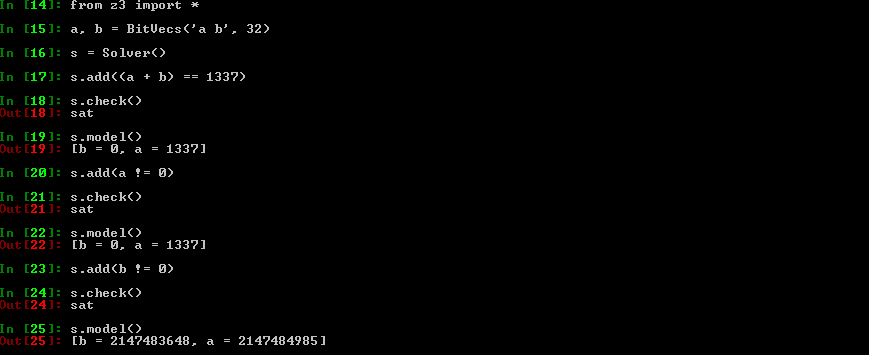## Z3py Hello-World: Explanations I

• BitVecs are basically arrays of 0 & 1's

• It is the type the closest from what your CPU handles

• A BitVec8 is basically the equivalent of an unsigned char variable in C

• A solver instance holds a set of constraints you want to apply

• Obviously, you can apply constraints only on Z3 variables: not on normal Python variables

## Z3py Hello-World: Explanations II

• BitVecs are not the only type available, you can also use: Ints, Bools, Arrays, etc.

• Note that Z3 integers are integers like in math: they are infinite ; as opposed to BitVecs

•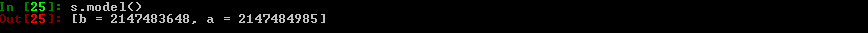• Why :-)?

• BitVecs do wrap, like CPU registers or C integers## Z3py Hello-World: Explanations III

• Important detail: Z3 data-types don't hold any signess information

• Operators do

• You have operators for signed & unsigned operations:

• Signed operators: <, <=, >, >=, /, %and, >>

• Unsigned operators: ULT, ULE, UGT, UGE, UDiv, URemand, LShR## Z3py tips: solve & Solver

• Maybe the most important function: this is the function that will answer your questions

• If solvable, it gives you a model (even if several exist) which is basically concrete values for your symbolic variables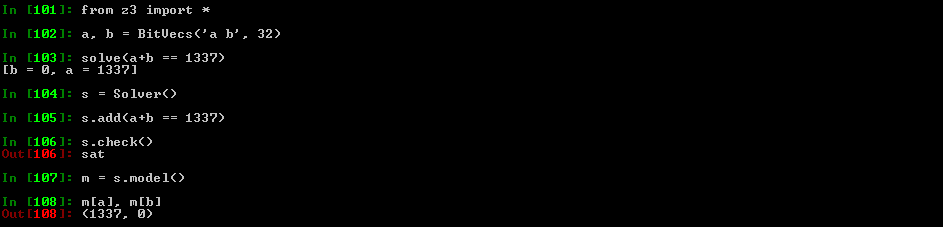## Z3py tips: Solver & backtracking points

• You can create backtracking points by using push and pop

• It basically saves the constraints you have set in the solver: it's a checkpoint

• As the name suggests, it's particularly useful when using backtracking algorithms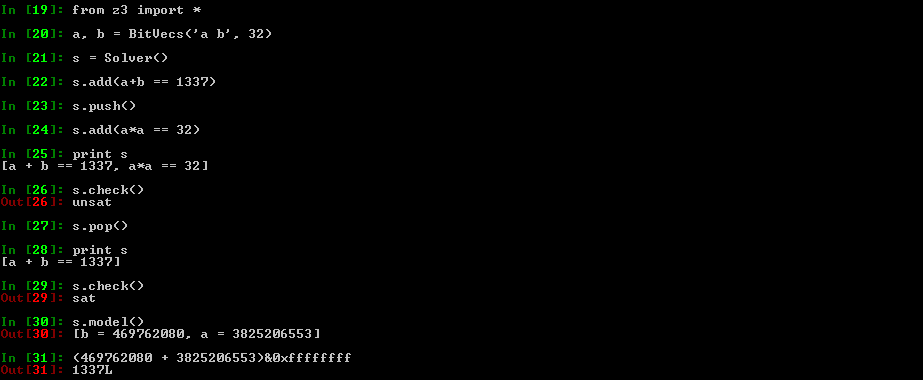## Z3py tips: simplify

• This function is something really important: as the name suggests it can simplify Z3 expressions

• Keep in mind it's not magic though: the returned expression does not have to be the most simplified expression (cf Breaking Kryptonite's Obfuscation)

• A lot of options can be enabled / disabled to help simplify to do a better job at simplifying ; call help_simplify()## Z3py tips: ZeroExt, SignExt

• Two useful functions when you deal with BitVecs

• As their names suggest, they basically zero or sign extend a BitVec

• Use case: zero extend a 32 bits BitVec into a 64 bits BitVec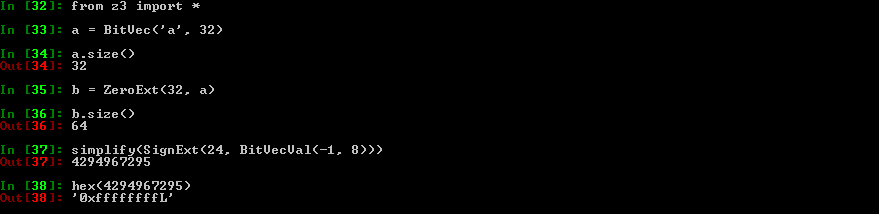## Z3py tips: Extract, Concat

• Another couple of useful functions:

• Extract: Extract some bits from a BitVec

• Concat: Concatenate BitVecs

• Z3 operators need operands of the same size ; Extract/Concat/ZeroSignExt can help you to meet this requirement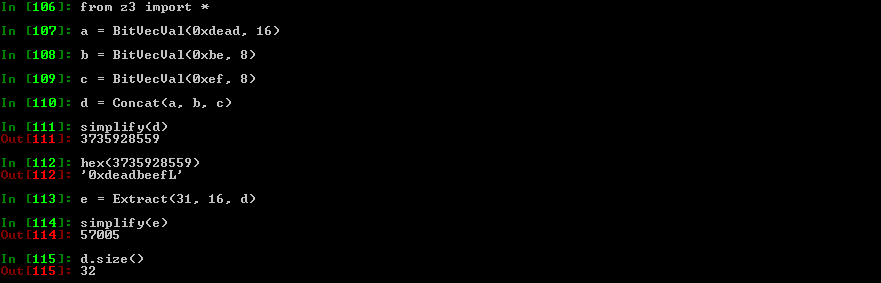## Z3py tips: RotateRight/Left

• Equivalent of x86's ROL/ROR instructions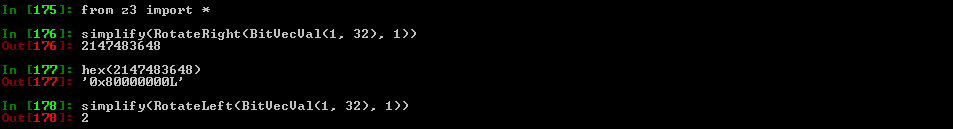## Z3py tips: prove

• Another quite cool & important function is prove

• The function basically checks the given equation is always true

• If not proven, Z3 will give you a counterexample

• Example: Let's prove that Concat works properly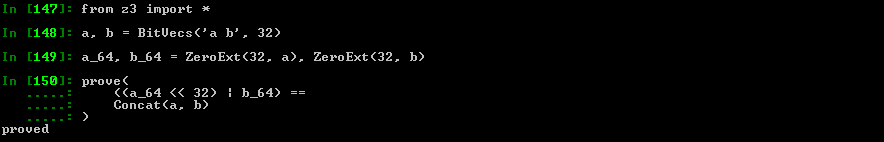## Z3py tips: prove example II

• More real example:

• Can we use ((a + b) < a) to know if there is an overflow when adding unsigned 32 bits integers a and b?

• Instead of storing the result in a 64 bits integer & checking that the result is not >= 0x100000000?

• proof_unsigned_integer_overflow_chech.py

```                            ```def does_overflow_custom_check(a, b):
return If(
ULT((a + b), a), # ULT = < unsigned
True,
False
)

def does_overflow_check(a, b):
a_64 = ZeroExt(32, a)
b_64 = ZeroExt(32, b)
return If(
UGE((a_64 + b_64), BitVecVal(0x100000000, 64)), # UGE = >= unsigned
True,
False
)
x, y = BitVecs('a b', 32)

prove(
does_overflow_check(x, y) ==
does_overflow_custom_check(x, y)
)
```
```

## Z3py tips: prove example II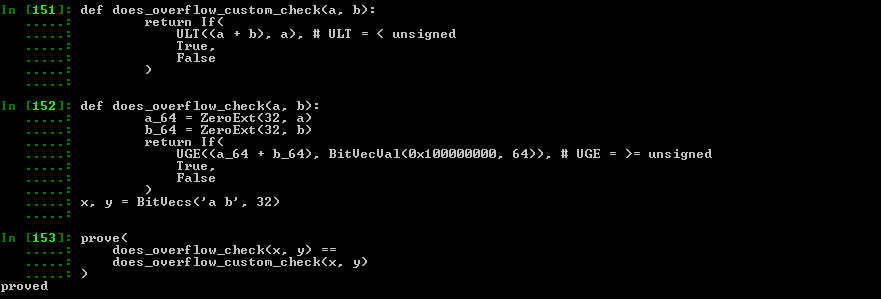## Z3py tips: Arrays

• Quite handy to simulate the memory in a symbolic way

• Z3 will use a brute-force approach, it will essentially try all possible combinations. It will not manage to find the "smart" proof that we (as humans) immediately see.

• You can access the array content with [] or Select, and write to it via Store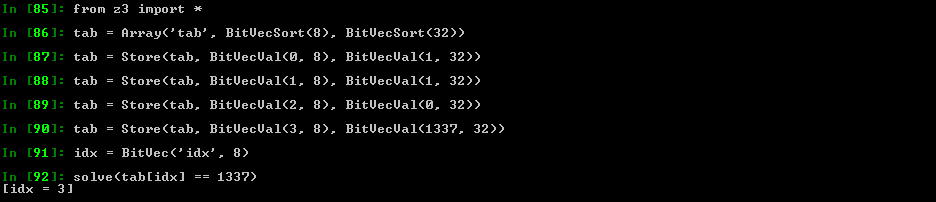## Z3py tips: substitute

• This function allows you to substitute variables by whatever you want in an expression

• You can replace a symbolic variable by a concrete value

• Or the way around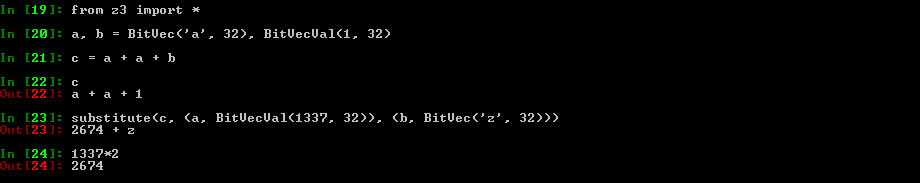## Z3Py tips: And/Or/Distinct

• Equivalent to Python's and & or

• They are really nice to use: you can give them an array of expressions, or inlined expressions

• A lot of time you need to express the following: "I want all my symbolic variables to be different"

• You can do it manually via And(x1 != x2, x2 != x3, x1 != x3)

• Or you can use Distinct(x1, x2, x3) which is way cleaner## Z3Py tips: If

• It is sometimes useful, but it's not like Python's if & else

• Different how? Not really easy to explain

• Here is a C code / Z3 example:

```                                    ```
unsigned int a, b;
if((a + b) < 100)
{
a += 100;
b -= 10;
}
else
{
a = 1337;
b += 1000;
}
```
```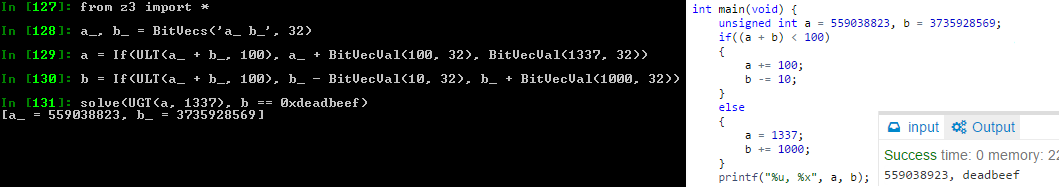## Z3Py tips: Visiting Z3 expressions

• You may need to inspect & visit the AST of a Z3 expression

• Useful for transformation, conversion, etc.

• The expression is a tree

• e.arg(x) gets you the x th argument in the expression

• e.num_args() gives you the number of argument

• Check z3topy.py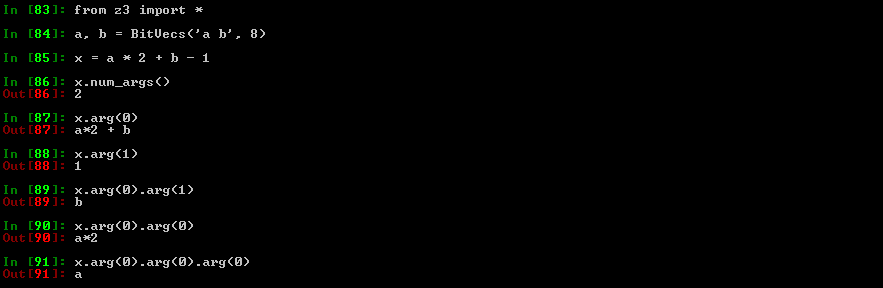## Z3Py toys: graph coloring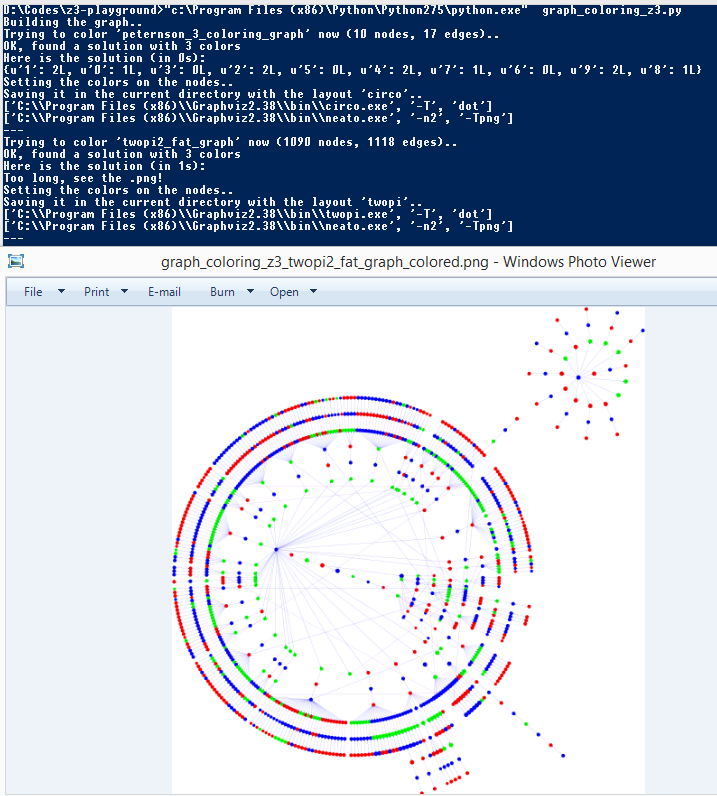## Z3Py toys: NQueens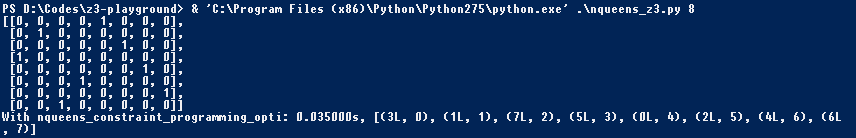## Z3Py toys: Mojette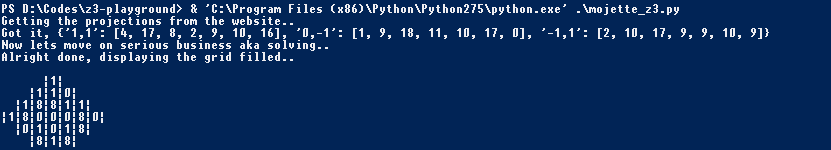## Z3Py toys: Magic square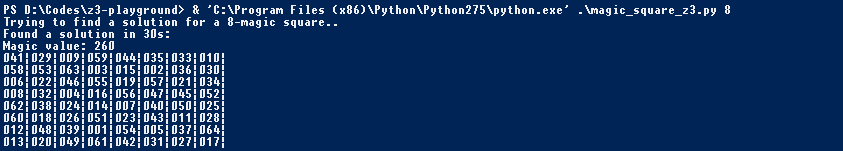## Conclusion

• Z3 is really powerful, use it!

• There are loads of advanced features that I don't use / I don't know how to use:

• Tactics / Strategies / Subgoals?

• Functions?

• Patterns?

• Data-types?

• Quantifiers?

• If you don't like Z3's API and / or its features try out another one:

# Symbolic execution 101

## Symbolic execution: definition with words

• Symbolic execution involves computation of a mathematical expression that represents the logic within a program. It can be thought of as an algebra designed to express computation.
Richard @richinseattle Johnson, NSC 2014
• An analogy I like to give between concrete / symbolic execution and mathematical functions:
• Concrete execution would be to know that f(3) = 19
• Symbolic execution would be to know that f(x) = x**2 + 10
• Symbolic execution expresses every concrete executions possible

## Symbolic execution: definition with words II

• So does that mean I have to recode a virtual CPU with the semantic of every instructions?
• Nope ; see it as a recipe. Take shortcuts to make your life easier:
• I don't need to handle branches
• I only need to handle 4 different instructions
• Think about the granularity you want: a basic block, a function, a library, an entire program
• Why not symbolically execute intermediate representation code instead of assembly?

## Symbolic execution: definition with code

• Let's imagine this code

```                                ```void hello(unsigned char c)
{
unsigned char win;
unsigned int a = c;
if(a >= 0 && a < 0xbaad)
win = 1;
else
win = 0;
}
```
```
• Concrete:

• c = 0xf0
• a = 0x000000f0

• win = 0
• Symbolic:

• c is an input sym var
• a_0 = ZeroExt(24, c)
• a = a_0*2 + 0xdeadbeef

• win = If(And(UGE(a, 0), ULT(a, 0xbaad)), 1, 0)

# Practical reverse-engineering

## Breaking weak hash function: hash collisions

• Original blog-post by James '@tiraniddo' Forshaw: Generating hash collisions

• The problem in details ; bear with me:
• Goals
• s = "abc\0" + SUFFIX
• H(s) == H("xyz")
• strcmp(s, "abc") == 0
• Definitions
• SUFFIX is a string fully controlled: even non-ASCII characters are allowed
• H is a hashing function
• Let's even find an ASCII printable SUFFIX!

## Breaking weak hash function: hash collisions

• Modeling C concrete strings
• Sequence of BitVecVal8
• ```                                    ```def str_to_BitVecVals8(s):
return map(
lambda x: BitVecVal(ord(x), 8),
list(s)
)
```
```
• Modeling ASCII-printable C symbolic strings
• Sequence of BitVec8
• ```                                    ```def ascii_printable(x):
return And(0x20 <= x, x <= 0x7f)

def generate_ascii_printable_string(base_name, size, solver):
bytes = [BitVec('%s%d' % (base_name, i), 8) for i in range(size)]
return bytes
```
```

## Breaking weak hash function: hash collisions

• Modeling H
• ```                                ```def H(input_bytes):
h = 0
for byte in input_bytes:
h = h * 31 + ZeroExt(24, byte)
return h
```
```

## Breaking weak hash function: hash collisions

• Putting it all together:
• ```                                ```def collide(target_str, base_str):
size_suffix = 7
s = Solver()
res = str_to_BitVecVals8(base_str) + [BitVecVal(0, 8)] + generate_ascii_printable_string('res', size_suffix, s)
if s.check() == sat:
x = s.model()
return base_str + '\x00' + ''.join(chr(x[i].as_long()) for i in res[-size_suffix:])
raise Exception('Unsat!')

def main(argc, argv):
a = 'xyz'
b = 'abc'
c = collide(a, b)
print 'c = %r' % c
print 'H(%r) == H(%r)' % (a, c)
print 'strcmp(%r, %r) = 0' % (c, b)
```
```

## Breaking weak hash function: hash collisions

• Job done!
•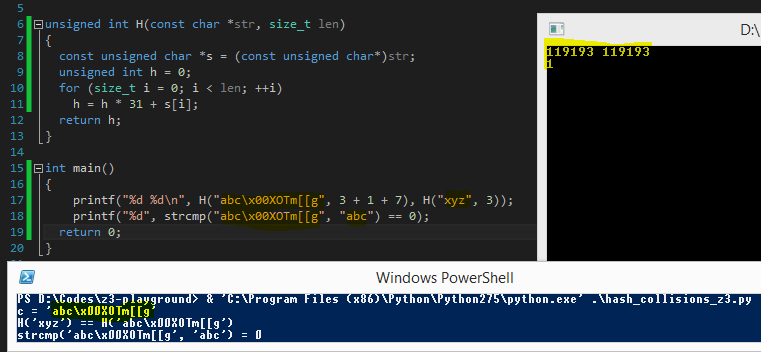• No brute-force, elegant solution: didn't even thought at how we could have "reversed" H, Z3 did it for us
• Full code is here: hash_collisions_z3.py

## Breaking conservative semantic obfuscation: Kryptonite

• Kryptonite is an LLVM optimization pass that adds semantic-preserving obfuscation for arithmetic operations

• PoC I've written up in "Obfuscation of steel: meet my Kryptonite" back in 2013

• Doing the transformation at the LLVM IR level allows you to support every LLVM back-end architecture: ARM, x86, x64 & a lot of others

• It also means you can write your code in every languages supported by LLVM

• And obviously, you can output binaries in whatever executable formats LLVM supports: Elf, Mach-o, PE

• Check out O-LLVM if you are interested in this field

• The semantic of the program is preserved: the assembly is just a bit disturbing

## Breaking conservative semantic obfuscation: Kryptonite

```                            ```
unsigned int add(unsigned int a, unsigned int b)
{
return a + b;
}

int main(int argc, char* argv[])
{
if(argc != 3)
return 0;

return 1;
}```
```
```                            ```\$ wget https://raw.github.com/0vercl0k/stuffz/master/llvm-funz/kryptonite/llvm-functionpass-kryptonite-obfuscater.cpp
\$ clang++ llvm-functionpass-kryptonite-obfuscater.cpp `llvm-config --cxxflags --ldflags --libs core` -shared -o llvm-functionpass-kryptonite-obfuscater.so
```
```

## Breaking conservative semantic obfuscation: Kryptonite

```                            ```PS C:\Users\0vercl0k> dir D:\Codes\llvm-funz\kryptonite-add
Length Name
------ ----
```
```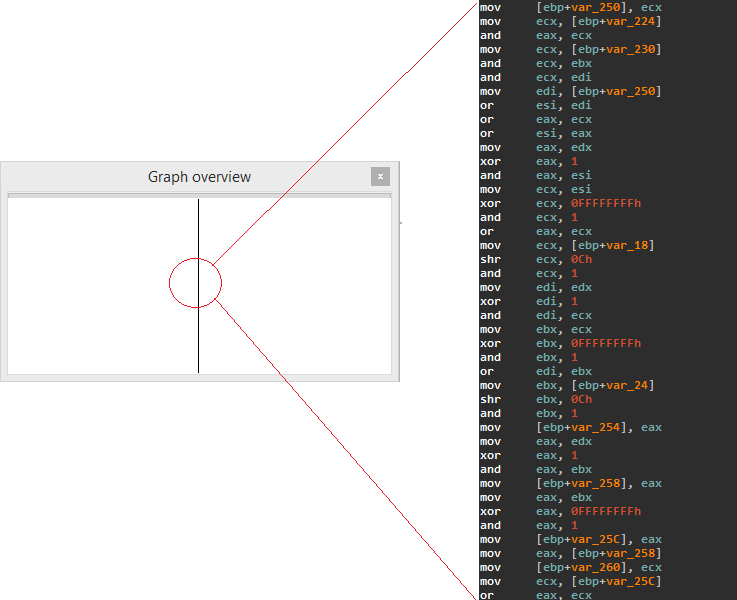## Breaking conservative semantic obfuscation: Kryptonite

• The context

• We want to attack a single basic block

• If you look closer at the previous slide, only a subset of x86's instructions are used

• Their semantics are easy to implement

• We don't need to handle branches, nor EFLAGS

• We don't know the number of input symbolic variables

• Heuristic: Every time we read from an uninitialized location, we treat it as an input variable

• The plan

• Symbolically execute the basic block

• To do so you need a virtual environment: both CPU & memory

• For us, virtual CPU = bunch of registers that can either hold symbolic variables or concrete values

## Breaking conservative semantic obfuscation: Kryptonite

• A disassembler class

• We need to parse the code to execute: IDAPython, string manipulation

• A virtual CPU: the core of the program

• Use the disassembler component & implement the instruction semantics

• Also simulates memory

• Keep track of the (simplified) equations

```                            ```Launching the engine..
Trying to read a non-initialized area, we got a new symbolic variable: arg0
Trying to read a non-initialized area, we got a new symbolic variable: arg1
Done, retrieving the equation in EAX, and simplifying..
```
```

## Breaking conservative semantic obfuscation: Kryptonite

```                            ```EAX=(~(Concat(2147483647, Extract(0, 0, arg1)) |
Concat(2147483647, ~Extract(0, 0, arg0)) |
4294967294) |
~(Concat(2147483647, ~Extract(0, 0, arg1)) |
Concat(2147483647, Extract(0, 0, arg0)) |
4294967294)) +
Concat(~(Concat(1073741823, Extract(1, 1, arg1)) |
Concat(1073741823, ~Extract(1, 1, arg0)) |
Concat(1073741823,
~(~Extract(0, 0, arg1) |
~Extract(0, 0, arg0)))) |
~(Concat(1073741823, ~Extract(1, 1, arg1)) |
Concat(1073741823, Extract(1, 1, arg0)) |
Concat(1073741823,
~(~Extract(0, 0, arg1) |
~Extract(0, 0, arg0)))) |
~(Concat(1073741823, Extract(1, 1, arg1)) |
Concat(1073741823, Extract(1, 1, arg0)) |
Concat(1073741823, ~Extract(0, 0, arg1)) |
Concat(1073741823, ~Extract(0, 0, arg0)) |
2147483646) |
~(Concat(1073741823, ~Extract(1, 1, arg1)) |
Concat(1073741823, ~Extract(1, 1, arg0)) |
Concat(1073741823, ~Extract(0, 0, arg1)) |
Concat(1073741823, ~Extract(0, 0, arg0)) |
2147483646),
0) +
...
```
```
• Not quite what I expected but as I mentioned before, simplification strategies are not magic ; complicated problem

## Breaking conservative semantic obfuscation: Kryptonite

• But we can eventually prove it is equivalent to arg0 + arg1 & then replace the expression with the simplified version right?

```                            ```
# The two expressions are equivalent ; we got a simplification!
if prove(Sum(self.sym_variables) == eq):
return Sum(self.sym_variables)

return eq

def get_reg_equation_simplified(self, reg):
eq = self.get_reg_equation(reg)
return eq
#[..]
print sym.get_reg_equation_simplified('eax')
```
```Launching the engine..
Trying to read a non-initialized area, we got a new symbolic variable: arg0
Trying to read a non-initialized area, we got a new symbolic variable: arg1
Done, retrieving the equation in EAX, and simplifying..
EAX=arg0 + arg1
```
```
Job done, more details here if you want

## Finding ROP gadgets with constraints

• The context

• ROP gadget = relatively small sequence of instructions that ends with a branching instruction

• Is "xor eax, eax ; ret" equals to "mov eax, 0xffffffff ; inc eax ; ret"?

• They are not stricly equal, nope

• Why? EFLAGS.AF for example

• What if we care only about the state of EAX after the gadget execution?

• In that case they are equivalent!

• The gadgets are small & they usually only use a small subset of x86 instructions: we don't really care about: SSE/MMX or crazy instructions

The problem

• To be able to compare gadgets, we need instruction semantics ; where do we get that for free?

## Finding ROP gadgets with constraints

• The solution

• Introducing amoco a Python framework for static program analysis developed by Axel '@bdcht' Tillequin

• Symbolic execution engine for x86, x64, ARM & instruction semantics

• Uses its own expression mechanism so far

• Most of important (for us) x86 instructions are implemented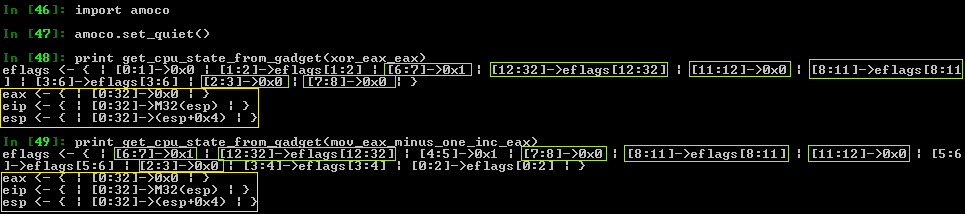## Finding ROP gadgets with constraints

• The plan

• Symbolically execute gadgets with amoco

• Because it uses its own "expression" classes, we need to sort of convert that in Z3

• @bdcht plans to integrate Z3 in his framework at some point

• No more magic ugly tricks to go from one to another

• Then we are free to compare the state of the two virtual CPUs

## Finding ROP gadgets with constraints

• What it looks like in the code:

• "I want EAX = EBX = 0 at the end of the gadget execution"

```                                                    ```
cpu_state_end_target.wants_register_equal('eax', 0)
cpu_state_end_target.wants_register_equal('ebx', 0)
```
```
• "I want ((ESP >= ESP + 1000) && (ESP < ESP + 2000)) && (EAX == 0)"

```                                                    ```
cpu_state_end_target.wants_register_greater_or_equal('esp', cpu.esp + 1000)
cpu_state_end_target.wants_register_lesser('esp', cpu.esp + 2000)
cpu_state_end_target.wants_register_equal('eax', 0)
```
```
• "I want EIP = ESP at the end of the gadget execution"

```                                                    ```
cpu_state_end_target.wants_register_equal('eip', 'esp')
```
```

## Finding ROP gadgets with constraints

• Example of cool results:

• "I want EAX = EBX = 0 at the end of the gadget execution"

```                                                    ```
xor eax, eax ; push eax ; mov ebx, eax ; ret
xor eax, eax ; xor ebx, ebx ; ret
```
```
• "I want ((ESP >= ESP + 1000) && (ESP < ESP + 2000)) && (EAX == 0)"

```                                                    ```
xor eax, eax ; add esp, 0x45c ; pop ebx ; pop esi ; pop edi ; pop ebp ; ret
```
```
• "I want EIP = ESP at the end of the gadget execution"

```                                                    ```
add dword ptr [ebx], 2 ; push esp ; ret
jmp esp
pushad ; mov eax, 0xffffffff ; pop ebx ; pop esi ; pop edi ; ret
```
```

## Taming a wild MIPS binary

• The context

• NoSuchCon 2014 crack-me (the first of the three challenges) coded by @elvanderb

• ELF MIPS binary protected by nanomites

• Father debugs the son ; Father's code is called each time the son executes break (equivalent of int3)

• Father modifies the son's CPU context to obfuscate the execution flow: the son won't be executed as it appears in IDA

• The protection scheme:

• Serial of 24 bytes

• win = memcmp(F(serial_entered), '[ Synacktiv + NSC = <3 ]') == 0

## Taming a wild MIPS binary

• Long story short:

• The father uses an algorithm to know where it has to redirect his son

• Once we have it, we know in which order the son is going to execute its code

• The son uses an algorithm to "decrypt" the serial (6 DWORDs)

• Each DWORD is modified in place ; each DWORD is modified in a different way thanks to the father

• Once we figure out the way each DWORD is modified, we can reverse it & break the challenge

## Taming a wild MIPS binary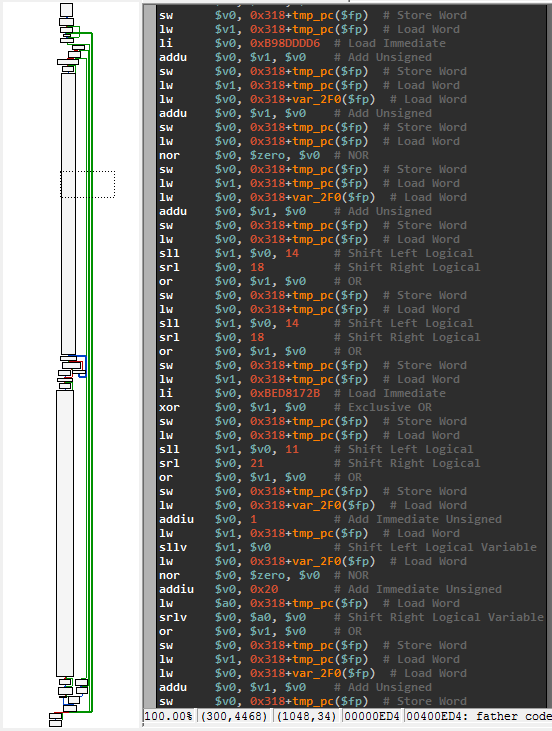## Taming a wild MIPS binary

• The plan:

• Symbolicly execute the three or four big basic blocks we need

• Then ask Z3 to find the input required to have F(serial) = '[ Synacktiv + NSC = <3 ]'

• We don't need branches & only need to implement < 20 instruction in our virtual CPU

• Most instructions are about basic mathematical computations: easy to implement with Z3

• Memory simulation with a simple array

• We can use our code both as a simplistic MIPS emulator (with concrete values)..

• ..or as a symbolic execution engine (with symbolic variables)

• All of that in less than 500 lines of Python

## Taming a wild MIPS binary

• The plan:

• Once we have an engine working:

• We can use it to recover the father algorithm

• Execution flow deobfuscation: we have now the code of the son unscrambled / clean

• We can use it to recover the son algorithm

• Ask Z3 the correct input values & job done!

• All of that in less than 300 lines of Python

## Taming a wild MIPS binary

• What the first DWORD looks after all the modification:

```                            ```
; input_dword_0
(declare-fun a () (_ BitVec 32))
(let ((?x966 ((_ extract 31 30) (bvadd (_ bv1169698645 32) (bvnot a)))))
(let ((?x991 ((_ extract 4 4) (bvadd (_ bv21 5) (concat (bvadd (_ bv5 3) (bvnot ((_ extract 2 0) a))) ?x966)))))
(let ((?x999 (bvnot ?x991)))
(let ((?x952 ((_ extract 15 5) (bvadd (_ bv35861 16) (concat (bvadd (_ bv12117 14) (bvnot ((_ extract 13 0) a))) ?x966)))))
(let ((?x1002 (concat ((_ extract 29 27) (bvadd (_ bv1169698645 32) (bvnot a))) (bvadd (_ bv95956821 27) (bvnot ((_ extract 26 0) a))) ?x966)))
(let ((?x980 (bvadd (_ bv3493170197 32) ?x1002)))
(let ((?x1003 (concat (bvnot (bvadd (_ bv5 4) (concat (bvadd (_ bv1 2) (bvnot ((_ extract 1 0) a))) ?x966))) (bvnot ((_ extract 31 17) ?x980)) (bvnot ((_ extract 16 16) ?x980)) (bvnot ?x952) ?x999)))
(let ((?x1008 (bvadd (_ bv422262738 32) ?x1003)))
(let ((?x1017 (concat (bvnot ((_ extract 26 17) ?x980)) (bvnot ((_ extract 16 16) ?x980)) (bvnot ?x952) ?x999)))
(let ((?x1018 (bvadd (_ bv2832338 23) ?x1017)))
(let ((?x1085 (concat (bvnot ((_ extract 22 21) ?x1018)) ((_ extract 20 17) ?x1018) (bvnot ((_ extract 16 16) ?x1018)) ((_ extract 15 15) ?x1018) (bvnot ((_ extract 14 14) ?x1018)) ((_ extract 13 12) ?x1018) (bvnot ((_ extract 11 11) ?x1018)) ((_ extract 10 10) ?x1018) (bvnot ((_ extract 9 7) ?x1018)) ((_ extract 6 6) ?x1018) (bvnot ((_ extract 5 5) ?x1018)) ((_ extract 4 4) ?x1018) (bvnot ((_ extract 3 1) ?x1018)) ?x999 ((_ extract 31 31) ?x1008) (bvnot ((_ extract 30 30) ?x1008)) ((_ extract 29 29) ?x1008) (bvnot ((_ extract 28 28) ?x1008)) ((_ extract 27 25) ?x1008) (bvnot ((_ extract 24 23) ?x1008)))))
(let ((?x1025 (concat (bvadd (_ bv12 4) (concat ((_ extract 26 25) ?x1008) (bvnot ((_ extract 24 23) ?x1008)))) ((_ extract 31 4) (bvadd (_ bv1338037900 32) ?x1085)))))
(let ((?x984 (bvnot ((_ extract 17 17) (bvadd (_ bv2036738909 32) ?x1025)))))
(let ((?x1001 (concat (bvnot (bvadd (_ bv11101 17) ((_ extract 20 4) (bvadd (_ bv1338037900 32) ?x1085)))) (bvnot ((_ extract 31 17) (bvadd (_ bv2036738909 32) ?x1025))))))
(let ((?x1010 (bvadd (_ bv3562860298 32) ?x1001)))
(let ((?x1004 (bvnot ((_ extract 1 1) ?x1010))))
(let ((?x1201 ((_ extract 3 3) (bvadd (_ bv6 5) (concat (bvnot ((_ extract 4 4) ?x1010)) ((_ extract 3 2) ?x1010) ?x1004 ?x984)))))
(let ((?x1196 ((_ extract 4 4) (bvadd (_ bv6 5) (concat (bvnot ((_ extract 4 4) ?x1010)) ((_ extract 3 2) ?x1010) ?x1004 ?x984)))))
(let ((?x1020 (concat ((_ extract 31 31) ?x1010) (bvnot ((_ extract 30 30) ?x1010)) ((_ extract 29 29) ?x1010) (bvnot ((_ extract 28 28) ?x1010)) ((_ extract 27 27) ?x1010) (bvnot ((_ extract 26 25) ?x1010)) ((_ extract 24 23) ?x1010) (bvnot ((_ extract 22 22) ?x1010)) ((_ extract 21 17) ?x1010) (bvnot ((_ extract 16 13) ?x1010)) ((_ extract 12 12) ?x1010) (bvnot ((_ extract 11 11) ?x1010)) ((_ extract 10 9) ?x1010) (bvnot ((_ extract 8 4) ?x1010)) ((_ extract 3 2) ?x1010) ?x1004 ?x984)))
(let ((?x1061 (bvadd (_ bv4035799430 32) ?x1020)))
(let ((?x1206 (concat (bvnot ((_ extract 2 2) (bvadd (_ bv6 3) (concat ((_ extract 2 2) ?x1010) ?x1004 ?x984)))) ((_ extract 1 1) (bvadd (_ bv6 3) (concat ((_ extract 2 2) ?x1010) ?x1004 ?x984))) ((_ extract 17 17) (bvadd (_ bv2036738909 32) ?x1025)) ((_ extract 31 30) ?x1061) (bvnot ((_ extract 29 29) ?x1061)) ((_ extract 28 27) ?x1061) (bvnot ((_ extract 26 25) ?x1061)) ((_ extract 24 22) ?x1061) (bvnot ((_ extract 21 21) ?x1061)) ((_ extract 20 16) ?x1061) (bvnot ((_ extract 15 15) ?x1061)) ((_ extract 14 12) ?x1061) (bvnot ((_ extract 11 9) ?x1061)) (bvnot ((_ extract 8 8) ?x1061)) ((_ extract 7 7) ?x1061) (bvnot ((_ extract 6 6) ?x1061)) (bvnot ((_ extract 5 5) ?x1061)) ?x1196 (bvnot ?x1201))))
(let ((?x1043 (bvadd (_ bv3499560052 32) ?x1206)))
(let ((?x1230 ((_ extract 14 13) ?x1043)))
(let ((?x1223 (concat (bvnot ((_ extract 12 12) ?x1043)) ((_ extract 11 11) ?x1043) (bvnot ((_ extract 10 10) ?x1043)) ((_ extract 9 9) ?x1043) (bvnot ((_ extract 8 7) ?x1043)) ((_ extract 6 6) ?x1043) (bvnot ((_ extract 5 5) ?x1043)) ((_ extract 4 3) ?x1043) (bvnot ((_ extract 2 2) ?x1043)) ((_ extract 1 1) ?x1043) (bvnot ?x1201) (bvnot ((_ extract 31 30) ?x1043)) ((_ extract 29 28) ?x1043) (bvnot ((_ extract 27 27) ?x1043)) ((_ extract 26 25) ?x1043) (bvnot ((_ extract 24 15) ?x1043)) ?x1230)))
(let ((?x1005 (bvadd (_ bv2171031339 32) ?x1223)))
(let ((?x1229 (concat (bvnot ((_ extract 14 13) ?x1005)) (bvnot ((_ extract 12 12) ?x1005)) (bvnot ((_ extract 11 11) (bvadd (_ bv3883 12) (concat (bvnot ((_ extract 24 15) ?x1043)) ?x1230)))) ((_ extract 10 8) (bvadd (_ bv3883 12) (concat (bvnot ((_ extract 24 15) ?x1043)) ?x1230))) (bvnot ((_ extract 7 5) (bvadd (_ bv3883 12) (concat (bvnot ((_ extract 24 15) ?x1043)) ?x1230)))) ((_ extract 4 3) (bvadd (_ bv3883 12) (concat (bvnot ((_ extract 24 15) ?x1043)) ?x1230))) (bvnot ((_ extract 2 1) (bvadd (_ bv3 3) (concat (bvnot ((_ extract 15 15) ?x1043)) ?x1230)))) (bvnot (bvadd (_ bv1 1) ((_ extract 13 13) ?x1043))) (bvnot ((_ extract 31 30) ?x1005)) ((_ extract 29 22) ?x1005) (bvnot ((_ extract 21 21) ?x1005)) ((_ extract 20 20) ?x1005) (bvnot ((_ extract 19 19) ?x1005)) ((_ extract 18 16) ?x1005) (bvnot ((_ extract 15 15) ?x1005)))))
(let ((?x1132 (concat ((_ extract 23 22) ?x1005) (bvnot ((_ extract 21 21) ?x1005)) ((_ extract 20 20) ?x1005) (bvnot ((_ extract 19 19) ?x1005)) ((_ extract 18 16) ?x1005) (bvnot ((_ extract 15 15) ?x1005)))))
(concat ((_ extract 28 9) (bvadd (_ bv2400525917 32) ?x1229)) (bvadd (_ bv93 9) ?x1132) ((_ extract 31 29) (bvadd (_ bv2400525917 32) ?x1229))))))))))))))))))))))))))))))
```
```

## Taming a wild MIPS binary

```                            ```
PS D:\Codes\NoSuchCon2014> python .\solve_nsc2014_step1_z3.py
==================================================
> Instantiating the symbolic execution engine..
> Generating dynamically the code of the son & reorganizing / cleaning it..
> Configuring the virtual environment..
> Running the code..
> Instantiating & configuring the solver..
> Solving..
> Constraints solvable, here are the 6 DWORDs:
a = 0xFE446223
b = 0xBA770149
c = 0x75BA5111
d = 0x78EA3635
e = 0xA9D6E85F
f = 0xCC26C5EF
> Serial: 322644EF941077AB1115AB575363AE87F58E6D9AFE5C62CC
==================================================

root@debian-mipsel:~# /home/user/crackmips 322644EF941077AB1115AB575363AE87F58E6D9AFE5C62CC
good job!
Next level is there: http://nsc2014.synacktiv.com:65480/oob4giekee4zaeW9/
```
```

## Conclusion

• Symbolic execution & theorem provers are really two useful / powerful tools for security / reverse-engineering tasks

• The deck of slides will be available online

• Feel free to reach out to me via email / twitter for any questions and / or feedbacks

• Big thanks to SecurityDay for having me :-)

• "My proof readers are awesome" (c) Rolf, 2014

## Shameless plug: Diary of a reverse-engineer

We are always looking for cool posts / articles, feel free to reach out to us!

## Questions?